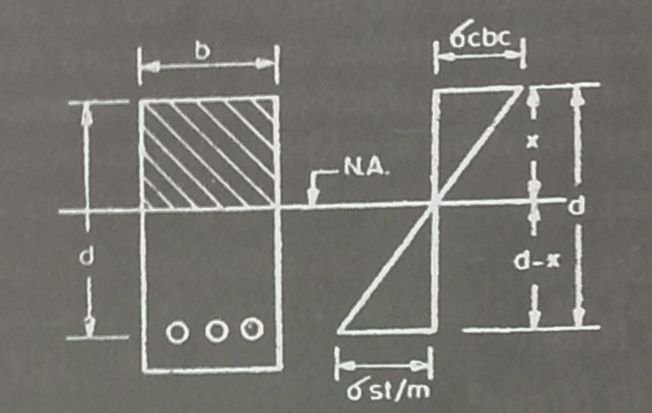## Design of Singly reinforced sections | Design Method 2

#### Guide to design of Singly reinforced Sections

For “Singly reinforced sections” article series, we have covered the following:

Now we will move on with our discussion on “2nd Design method” for the design of Singly reinforced Sections.
We will follow a simple three-step procedure for the design of singly reinforced sections.

#### Step One:

Given that:

• Dimensions of section (b and d)
• Area of tensile steel (Ast)
• Modular ratio (m)Stress-strain diagram

From the figure above, we can see that the neutral axis is situated at the centre of gravity of a given section. Therefore, the moments of area on either side are equal.

Read more